[an error occurred while processing this directive] Time-Distance Helioseismology
Time-Distance Helioseismology

## Time-Distance Helioseismology

The goal of time-distance helioseismology is to perform a time-distance analysis, where solar oscillations are characterized. The time-distance analysis measures directly the times taken by acoustic waves to travel from the solar surface to the bottom of the cavity and back. We refer to this type of travel as a raypath. This analysis also measures the distances as an angle at the surface between the surface reflections. In this analysis, time series at two separate points are crosscorrelated with all possible annuli as a function of travel time. From the crosscorrelations, time delays are measured. Later analysis takes the maps of time delays, inverts the data, and maps of solar interior properties are calculated -- in particular of the magnetic field, sound speed, flow speed and direction.

In the figure below, we show some raypaths below the Sun's surface. The rays go from the center of an annulus to annuli with different radii. The ray paths are curved because of the increasing sound speed with depth. The sound speed increases from 7 km/sec near the surface to 35 km/sec at 10 Mmeters depth. The measured travel times are sensitive to the component of velocity along the particular path.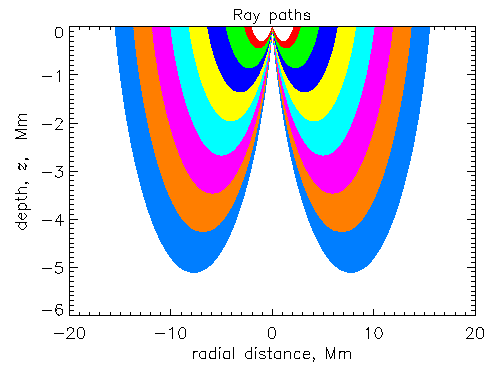A rule of thumb that we have found useful is that the horizontal distance between surface reflections is given approximately by pi multiplied by the depth of the turning point of the ray. This relation is exactly true for a polytrope and approximately true for a real solar model. Another useful rule of thumb is that the length of the ray is four times the depth.

For a fixed angular distance (in degrees), different waves travel to different depths in the Sun's convection zone. The shallower waves "bounce" more, while the deeper waves bounce less (and travel deeper).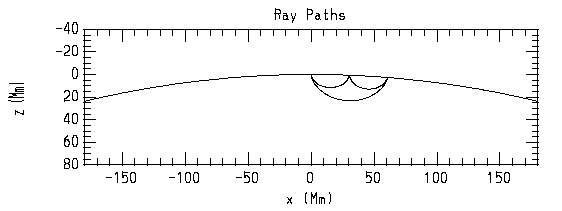The following diagram is representative of the output from Duvall's time-distance analysis.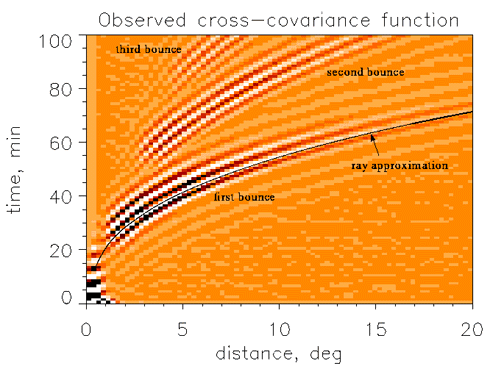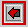SOHO SOI/MDI Results.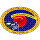The SOI Main Page.